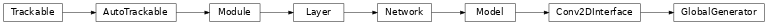# GlobalGenerator¶

Inheritance Diagramclass ashpy.models.convolutional.pix2pixhd.GlobalGenerator(input_res=512, min_res=64, initial_filters=64, filters_cap=512, channels=3, normalization_layer=<class 'ashpy.layers.instance_normalization.InstanceNormalization'>, non_linearity=<class 'tensorflow.python.keras.layers.advanced_activations.ReLU'>, num_resnet_blocks=9, kernel_size_resnet=3, kernel_size_front_back=7, num_internal_resnet_blocks=2)[source]

Global Generator from pix2pixHD paper.

• G1^F: Convolutional frontend (downsampling)
• G1^R: ResNet Block
• G1^B: Convolutional backend (upsampling)

Methods

 __init__([input_res, min_res, …]) Global Generator from Pix2PixHD. call(inputs[, training]) Call of the Pix2Pix HD model.

Attributes

 activity_regularizer Optional regularizer function for the output of this layer. dtype dynamic inbound_nodes Deprecated, do NOT use! Only for compatibility with external Keras. input Retrieves the input tensor(s) of a layer. input_mask Retrieves the input mask tensor(s) of a layer. input_shape Retrieves the input shape(s) of a layer. input_spec Gets the network’s input specs. layers losses Losses which are associated with this Layer. metrics Returns the model’s metrics added using compile, add_metric APIs. metrics_names Returns the model’s display labels for all outputs. name Returns the name of this module as passed or determined in the ctor. name_scope Returns a tf.name_scope instance for this class. non_trainable_variables non_trainable_weights outbound_nodes Deprecated, do NOT use! Only for compatibility with external Keras. output Retrieves the output tensor(s) of a layer. output_mask Retrieves the output mask tensor(s) of a layer. output_shape Retrieves the output shape(s) of a layer. run_eagerly Settable attribute indicating whether the model should run eagerly. sample_weights state_updates Returns the updates from all layers that are stateful. stateful submodules Sequence of all sub-modules. trainable trainable_variables Sequence of variables owned by this module and it’s submodules. trainable_weights updates variables Returns the list of all layer variables/weights. weights Returns the list of all layer variables/weights.
__init__(input_res=512, min_res=64, initial_filters=64, filters_cap=512, channels=3, normalization_layer=<class 'ashpy.layers.instance_normalization.InstanceNormalization'>, non_linearity=<class 'tensorflow.python.keras.layers.advanced_activations.ReLU'>, num_resnet_blocks=9, kernel_size_resnet=3, kernel_size_front_back=7, num_internal_resnet_blocks=2)[source]

Global Generator from Pix2PixHD.

Parameters: input_res (int) – Input Resolution. min_res (int) – Minimum resolution reached by the downsampling. initial_filters (int) – number of initial filters. filters_cap (int) – maximum number of filters. channels (int) – output channels. normalization_layer (tf.keras.layers.Layer) – normalization layer used by the global generator, can be Instance Norm, Layer Norm, Batch Norm. non_linearity (tf.keras.layers.Layer) – non linearity used in the global generator. num_resnet_blocks (int) – number of resnet blocks. kernel_size_resnet (int) – kernel size used in resnets conv layers. kernel_size_front_back (int) – kernel size used by the convolutional frontend and backend. num_internal_resnet_blocks (int) – number of blocks used by internal resnet.
call(inputs, training=True)[source]

Call of the Pix2Pix HD model.

Parameters: inputs – input tensor(s). training – If True training phase. Tuple – Generated images.